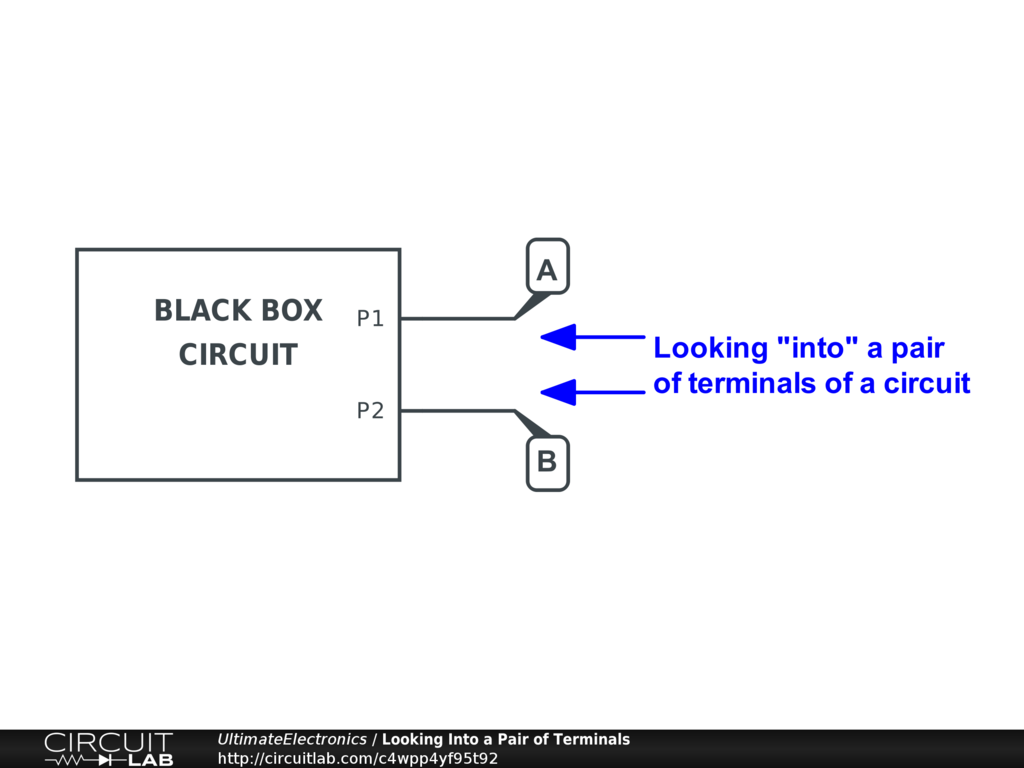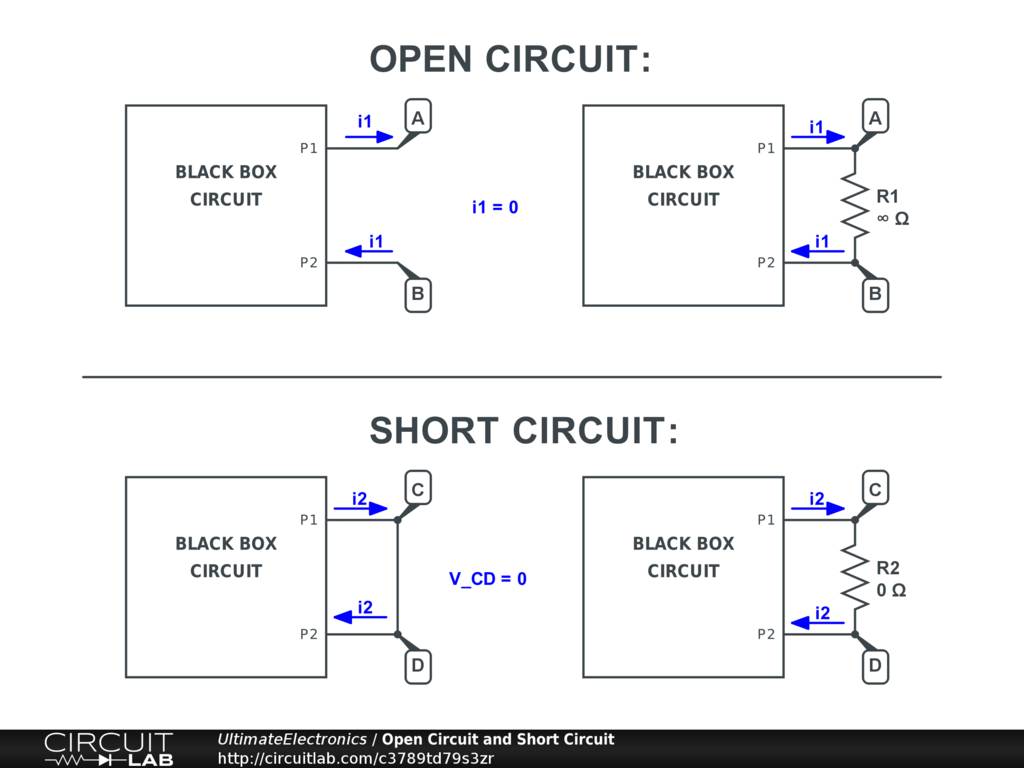Open Circuit and Short Circuit

Open circuit and short circuit are two special terms that represent opposite extremes of the resistance number line.

We can look at a circuit by looking at any pair of exposed terminals:In the context of any two terminals of a circuit:

A short circuit implies that the two terminals are externally connected with resistance $R=0$, the same as an ideal wire. This means there is zero voltage difference for any current value. (Note that real wires have non-zero resistance!)

An open circuit implies that the two terminals are points are externally disconnected, which is equivalent to a resistance $R=\infty$. This means that zero current can flow between the two terminals, regardless of any voltage difference. (Note that very high voltages can cause arcs of current to flow even over large air or vacuum gaps!)The concept of looking into two terminals of a circuit and looking at the behavior at these two extremes is a powerful one.

In both theory and practice, the word "externally" has no specific meaning. It's an arbitrary boundary to separate the "original" behavior of a circuit from the new behavior when we make certain modifications at any pair of nodes. This artificial boundary considers the rest of the circuit, the parts internal to the black box, to be unmodified. By making that assumption, we can make just one small change external to the black box and see its effect on the black box.

Use in Practical Measurement

An ideal voltmeter is open circuit. An open circuit is a limiting approximation for a real voltmeter, which will have some large (but not infinite) resistance.

An ideal ammeter is short circuit. A short circuit is a limiting approximation for a real ammeter, which will have some small (but not zero) resistance.

See the Multimeters & Measurements section for more.

Use in Theoretical Analysis

Just as a voltmeter and ammeter measure by connecting two probes to a circuit, theoretical analysis is often accomplished by looking at just two nodes of a circuit.

Open and short circuit provide two useful points on the V-I curve.

In particular:

• The open circuit voltage is the voltage difference measured between two terminals when no current is drawn or supplied.
• The short circuit current is the current that flows when the terminals are forced to have zero voltage difference.

We'll use these two values in Thevenin Equivalent and Norton Equivalent Circuits.

Use in Robust Design

In practical design, we'd like the circuits we build to survive both the normal conditions they are designed for, and some unusual conditions that happen occasionally but should not be allowed to cause permanent damage.

Open circuits happen even when undesired. For example, whenever something is disconnected or unplugged, we have an open circuit condition.

Short circuits also happen even when undesired. For example, if a connector momentarily shorts across two terminals as it's being inserted, or a tiny metal shaving ends up in the wrong place, we'll have a short circuit condition.

Where possible, we should design for open and short circuits to happen at various places within the circuit, especially at any exposed inputs and outputs. We should design such that any failures are temporary and/or recoverable, such as with a circuit breaker.

Use in Manufacturing

Intentional $R = 0 \ \Omega$ resistors (short circuit) are sometimes added to a printed circuit board because the designer wants the flexibility to change the value without having to redesign the printed circuit board later if they want to add some nonzero series resistance (or other series component) in the future.

Similarly, intentional jumper pads (open circuit) are sometimes added because the designer wants the flexibility to connect a section later, perhaps to add a parallel resistance.

Both of these can allow for flexible changes while sharing the same manufacturing overhead costs. This keeps unit costs low and avoids expensive redesign time.

What's Next

In the next section, Thevenin Equivalent and Norton Equivalent Circuits, we'll see how the two-terminal concept can be applied to making a simplified approximation of whatever is in the "black box circuit" labeled above.